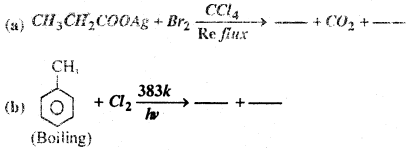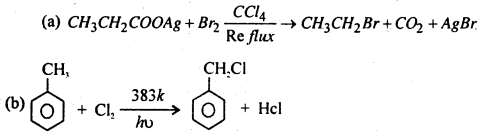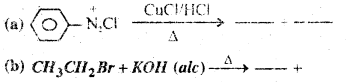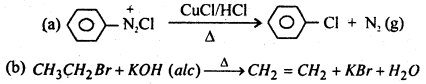# Bihar Board 12th Chemistry Important Questions Short Answer Type Part 4 in English

BSEB Bihar Board 12th Chemistry Important Questions Short Answer Type Part 4 are the best resource for students which helps in revision.

## Bihar Board 12th Chemistry Important Questions Short Answer Type Part 4 in English

Question 1.
Give reasons:
(i) Xenon does not form fluorides such as XeF3 and XeF5.
(ii) Out of noble gases, only xenon is known to form established chemical compounds.
(iii) Noble gases have very low boiling points.
(i) All the filled orbitals of Xe nave paired electrons. The promotion of one, two or third electrons from the 5p-filled orbitals to the 5d-vacant orbitals will give rise to two, four, six half-filled orbitals. Therefore, Xe can combine with even number of F atoms, but numbers. Thus, it cannot form XeF3 and XeF5

(ii) Except radon which is radioactive, Xe has the least ionization energy among noble gases hence it readily forms chemical compounds particularly with O2 and F2.

(iii) Noble gases are monoatomic. Their atoms are held together by weak dispersion form hence can be liquefied at very low temperatures. So, they have low boiling points.

Question 2.
Write the structures of the following species:
(i) H3PO2
(ii) H2SO5
(i)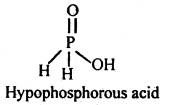(ii)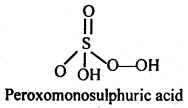Question 3.
Water is a Liquid White H2S is a gas. Explain.
Oxygen is more Electronegative than sulphur. H-Bond present between molecules of water, Therefore More water molecules get associated to form a bigger molecules. Consequently water exist as liquid at normal condition of temp. & pressure. But in H2S. H – Bond is absent, so H2S exist as gaseous state.

Question 4.
Write notes on – (i) Hund’s rule (ii)Aufbau principle.
(i) Hund’s rule- Hund’s governs the filling of electron in an orbital. According to this rule electron pairing in any orbital is not possible until all the available orbital of a given shell contain on electron each.

It is obvious from this rule that pairing must being with the introduction of the second electron in the s-subshell, the fouth electron in the p-subshell, the sixth electron in the d-subshell and the eight electron in the f-subshell.

For example, electronic configuration of nitrogen (at. no. = 7) is 1s2 2s2 2p3 since p orbitals contain only three electrons with must go in three p orbitals singly according to Hund’s rule. The orbitalwise configuration will be 1s2 2s2 2p1x 2p1y 2p2z . Electronic configuratin of oxygen (at. no. = 8) is 1s2 2s2 2p4. Here pairing of electron in p-subshell starts with the introduction of the fourth electron in it. Hence orbital wise configuration will be 1s2 2s2 2p2x 2p1y 2p1z. Similarly electronic configuration of F (at. no. =9) will be 1s2 2s2 2p2x 2p2y 2p1z and that of Ne (at. no. 10) is 1s2 2s2 2p2x 2p2y 2p2z.

Three p orbital are of equal energy but are concentrated indifferent region of space at right angles to each other. Electrons cause minimise the repulsive forces between themselves by occupying different orbitals and having parallel spins. This explains their preference to enter different orbitals as long as possbile.

(ii) Aufbau principle- Aufbau, a German expression pronounced as ‘of bow’ means building up. According to this principle, orbitals are filled in the order of increasing energy. In other words the orbital with a lower energy is filled up first before the filling of the orbital with higher energy commences.

As working rule a new electron enters the orbital for which (n + l) is minimum where n is principal quantum number and l-is azimuthal quantum number. When (n + l) has the same value for two or more orbitals, the new electron enters the orbital for which n is the minimum.

(n + l) values for various orbitals are as follows.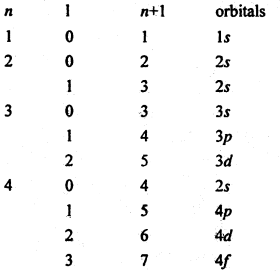using this rule the most usual sequence in which the electrons occupy various orbitals is seen to be 1s → 2s → 2p → 3s → 3p → 4s → 3d → 4p → 5s → 4d → 5p → 6s → 4f → 5d → 6p → 7s → 5f → 6d → 7p etc.
This, many expressed diagrammatically as follows: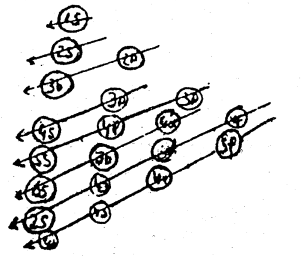For example, whether a new electron will go to 2p or 3s. This can be predicted, with the help of this rule, that the new electron will 2p sub-shell because for this sub-shell, n is 2 where as for 3s it is 3, though the values of (n + l) for both sub-shells is 3.

Question 5.
Discuss the (i) Bronsted-Lownyles concept and (ii) Lewis’s concept of acids and bases.
(i) (A) Bronsted-Lowry’s concept of acids and bases- A general definition of acids and bases was given independently by Lowry and Bronsted in 1973, according to which an acid is a compound or ion which gives up a proton to a base and a base is a compound or ion which accepts a proton from an acid. This relationship can be expressed in general as.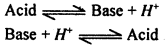That is for every acid there exists a base which is produced when the acid loose it proton. The acid and base so related are said to be conjugate to one another. Thus for acetic acid the acetate ion is evidently a base. The
CH3COOH ⇌ CH3COO + H+
Proton released in likely to be associated with the solvent molecule and the ionisation can be expressed in case water solvent.
CH3 COOH+ H2O ⇌ CH3 COO + H3O+

The acetic acid and acetate ion are then said to be conjugate acid-base pair. According to this definition the term acid includes not only molecules such as HCl, H2SO4, HNO3, etc but a great many species, molecular as well as ionic. In fact, water undergoes self ionization to a limited extent as :
H2O +H2O ⇌ H3O+ + OH
In the above equation, H3O+ is solvated proton or hydronium ion. Since proton has enormous affinity for electron, it is invariably solvated in water. In the self ionization some water molecules behave as acids and an equal and bases. A general definition of acids and bases number as bases. When acid dissolves in water it releases protons which are solvated to produce higher concentration of hydrimuim ions. For example, HCl ionises in water it release protons which are solvated to produce, higher concentration of hydronium ions. For example, HCl ionises in water almost completely according to the following equation-
HCl + H2O ⇌ H3O+ + Cl

Thus by definition NH4+ and HSO4 are also acids in aqueous media since they dissociate in water as :
NH4+ +H2O ⇌ NH3 + H3O+
HSO4 +H2O ⇌ SO4 + H3O+
SO4 and NH3 molecule are conjugate bases of the acids, HSO4 and NH4+respectively.

Following may be recognised as acids and bases in aqueous media :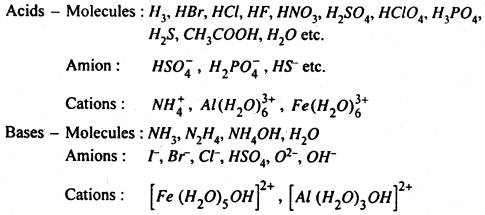The above discussion of acids and bases may be summarised as :
(i) A substance will be able to show its acidic character only if another substance is capable of accepting a proton. For example, HCl or CH3COOH in benzene solvent will not show the acidic properties because benzene does not take up protons. Thus water is capable of accepting proton then HCl or CH3COOH will ionised into it.

(ii) The proton H+ in aqueous solution is always hydrated i.e. H3O+ which is known as hydronium ion due to its resemblence with ammonium (NH4).

(iii) Acids and bases are not only the molecules but even ions may act like acid and bases. As given above Cl, HSO4, NO3, behave as a base because they can accept a proton.

(ii) Lewis acids and Bases- A more general acid-base concept was given by Lewis in 1923, who tried to receive the weakness of Lowry and Bronsted theory. According to him. “An acid is a substance (molecule, redical or ion which can accept a pair of electrons to form a co-ordinate compound and base is a substance which can furnish this pair of electron to form a co-ordinate compound.”

The above definition may be said in this way also that an acid is electron acceptor which a base is electron donner. In another way, it may be said Lewis base are the substances having unshared pair of electron while Lewis acids are the substance having empty orbitals in which the electron pair as given by the base may be accomodated. For example,
NH3 + BF3 → [H3N → BF3]

(ii) Reaction between calcium oxide and sulphur trioxide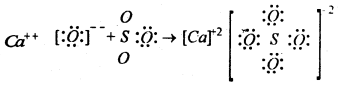In the above examples NH3 forms a bond using the unoccupied orbital of the boron atom in BF3. Similarly CaO behaves as Lewis base and SO3 behave as Lewis acid. Thus ammonia organic amines, ethers, alcohols, hydroxy ions, calcium Oxide etc. Which are capable of donating one lone pair of electrons through formation of co-ordinate bonds are called bases or Lewis bases. Compounds like, BF3, BCl3, Alcl3, SO3, SiF4 etc. are all capable of accepting one or more lone pairs of electrons through formation of co-ordinate bonds are called acids or Lewis acids.

A Lewis acid is often described as an electrophilic reagent (electron loving) and a base as a nucleophilic reagent. These terms are, however, more common to mechanism in organic chemistry.

The broadness of Lewis approach can be realised by examining the following three types of reactions :
(i) the acceptor has got less than a full acitet,
BF3 + : NH3 → H3NBF3
(ii) the acceptor has got incomplete orbitals.
Ag+ + 2NH3 → Ag (:NH3)2
and (iii) the acceptor has got a double bond.
O = C = O + H2O : → CO2 : H2O
Or, H2CO3

Lewis extended his concept of acids and bases further to define an acid as a substance which can accept a negative ion and a base as a substance which can provide a negative ion as exemplified in the following reaction,
SO2 + Ca2+ O2 → Ca2+ + SO32-
Thus SO2 is an acid and CaO is a base.

Unfortunately Lewis definition is so general that it includes reactions which are often more usefully considered from different stand points. For example, the following acid-base reactions can be better described in terms of complex formation and oxidation-reduction respectively.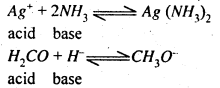Question 6.
Describe an applications of Kohlrausch’s law.
Following are the applications of Kohlrausch’s law :
(a) In Calculating the molar conductivity of a weak electrolyte at infinite dilution. Kohlrausch’s law is helpful in determining the limiting molar conductivities if weak electrolytes. The molar conductivity at infinite delusion $$\left(\wedge_{\mu}^{\infty}\right)$$ form weak electrolytes cannot be obtained directly by the extrapolation of plot of Am versus $$\sqrt{C}$$, But this can be easily calculated with the help of kohlrausch’s law.

For example, the value of $$\wedge_{m}^{\infty}$$ for acetic acid (CH3COOH) can be calculated from the knowledge of the molar conductivities at infinite dilution of strong electrolytes like CH3COONa, HCl and NaCl as follows: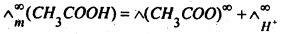Now add and subtract $$\lambda_{\left(\mathrm{Na}^{+}\right)}^{\infty}$$ and $$\lambda_{C l^{-}}^{\infty}$$ to the expression on right hand side and rearrange.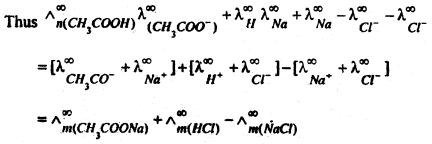(b) Calculation of degree of dissociation of weak electrolyte : Molar conductivity of an electrolyte depends upon its degree of dissociation. Higher the degree of dissociation, higher is the molar conductivity. As dilution increases the degree of dissociation of weak electrolyte also increases and consequently molar conductivity of electrolyte increases. At infinite dilution mola conductivity becomes maximum because degree of dissociation approach unity.

Thus if $$\wedge_{m}^{r}$$ = molar conductivity of solution at any concentration
$$\wedge_{m}^{\infty}$$ = molar conductivity at infinite dilution
α = degree of dissociation
α = $$\frac{\wedge_{m}^{r}}{\wedge_{m}^{\infty}}$$

Question 7.
Physical adsorption: When particles of the adsorbate and held to the surface of the adsorbent by weak forces such as Vande Waals force the adsorption is called physical adsorption of physisorption.

Chemical adsorption: When the molecular of the adsorbate are held to the surface of adsorbent by the chemical forces, the adsorption is called chemical adsorption. In case a chemical reaction occurs between the adsorbed molecules and the molecules or atoms of adsorbent. It is also known as chemisorption.

Difference between physical and chemical adsorption

1. Enthalpy of adsorption usually is the order of 20-40 kJ mol-1
2. Moles of adsorbate and adsorbent are held by Van der Waals interactions.
3. It usually takes place at low temperature and decreases with increasing temperature.
4. It is not very specific i.e. all gases are adsorbed at all solids to some, extent.
5. Multi-molecular layers may be formed on the adsorbent.
6. It does not require any activation energy.
7. The amount of adsorbed in related to the case of liquefaction of the gas.
8. It is reversible in nature.

1. Enthalpy of adsorption is of the order 40-400 kJ mole-1
3. It takes place are relatively high temperatures.
4. It is highly specific and takes place when there is some possibility of compound formation between the adsorbent molecules
5. Usually mono-molecular layer is formed on the adsorbent.
6. It requires activation energy
7. There is no such correlation.
8. It is irreversible in nature.

Question 8.
Write IUPAC Name of following Compounds.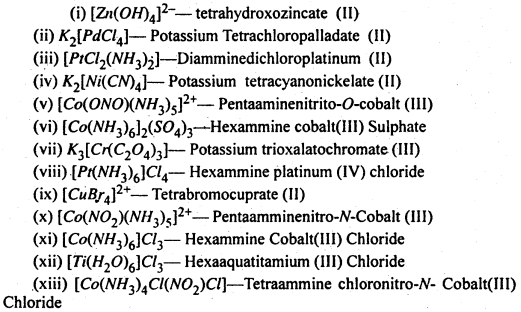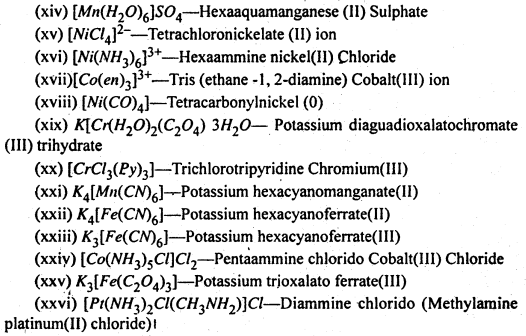Question 9.
Explain the following terms with suitable examples :
(i) Schottky defect, (ii) Frenkel defect, (iii) Interstitials.
(i) Schottky defect : If in an ionic crystal of the type A+B equal number of cations and anions are missing from their lattice sites so that the electrical neutrality is maintained, it is called Schottky defect.

The Schottky defects are shown by those compounds which have (a) high coordination number, (b) small differences in the size of cations and anions. A few examples are NaCl, KBr, CsCl, KCl etc. As number of ions decrease hence the density decrease because volume remains constant due to this defect.

(ii) Frenkel defect: In the ionic compound of the type A+B if an ion is missing from its lattice site, causing a vacancy or a hole there, and it occupies the interstial site, the electrical neutrality as well as the stoichiometry of the compound are maintained. This type of defect is called Frenkel defects.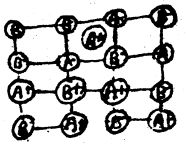Since cations are usually smaller, it is more common to find the cations occupying interstitial sites. The compounds which exhibit Ferenkel defect have (a) lower coordination number (b) large difference in the v size of cations and anions.

It is found in silver halides due to small size of Ag+ ion. Since no ions are missing from the crystal as a whole, density of the solid remains unchanged.

(iii) Interstitials : Atoms or ions which occupy the vacant interstial sites are called interstitials. The formation of interstitials depends upon the size of the atom or ion as it should be able to it in the void. The defeat that arises due to the presence of extra cations in the interstitial sites is called interstitial defect. Frenkel defect is a hybrid type of defect as it is a combination of schottky and intertidal defects.

Question 10.
Assign reasons for the following :
(a) Assign reasons for the following :
(i) The acid strengths of acids increase in the order.
HF < HCl < HBr < HI
(ii) Draw the structures of the following compounds :
(i) SF4 (ii) XeF2
(i) This is due to decrease in the bond dissociation energy of H-X on moving top to bottom in a group.
(ii)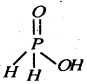Since it contains only one ionizable H-atom which is present as OH group, therefore, it behave as a monoprotic acid.

(b) (i) SF4 : sp3d hybridisation
b.p. = 4
Number of electrons pairs = 5
l.p. = 1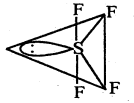(ii) XeF2 : sp3d hybridisation
b. p. = 4
Number of electrons pairs = 5
l.p. = 1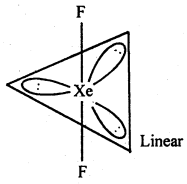Question 11.
Lithium Form b.c.c. crystal. Calculate the atomic radius, of Lithium if length of the side of unit cell of Lithium is 351 PM.
Given that a = 351 PM,
Suppose Atomic radius of Lithium is r
We know that Relation between Radius and side length of B.C.C. unit cell is: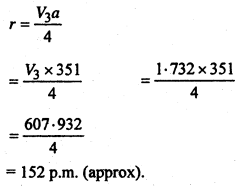Question 12.
Fill in the Blanks-